##### PostgreSQL CURRENT_TIMESTAMP Function With Examples

PostgreSQL offers multiple date/time functions, such as CURRENT_DATE, NOW(), EXTRACT(), CURRENT_TIMESTAMP, etc. If we talk about the CURRENT_TIMESTAMP function, it retrieves the current date, time, and timezone when a transaction starts.

This write-up will present a thorough overview of the Postgres CURRENT_TIMESTAMP function with examples. So, let’s begin.

How to Use CURRENT_TIMESTAMP Function in PostgreSQL?

Firstly, let’s understand the syntax of the CURRENT_TIMESTAMP function:

`CURRENT_TIMESTAMP(<precision>);`

Here, “precision” is an optional parameter used to round the “seconds” fields up to specific fractional digits. When the precision parameter is omitted, a TIMESTAMP will be returned with a timezone along with full fractional seconds precision.

Now, we will implement the CURRENT_TIMESTAMP function practically to get a better understanding:

Example #1: What Does CURRENT_TIMESTAMP Function Return?

Run the below statement to understand the working of the CURRENT_TIMESTAMP function:

`SELECT CURRENT_TIMESTAMP;`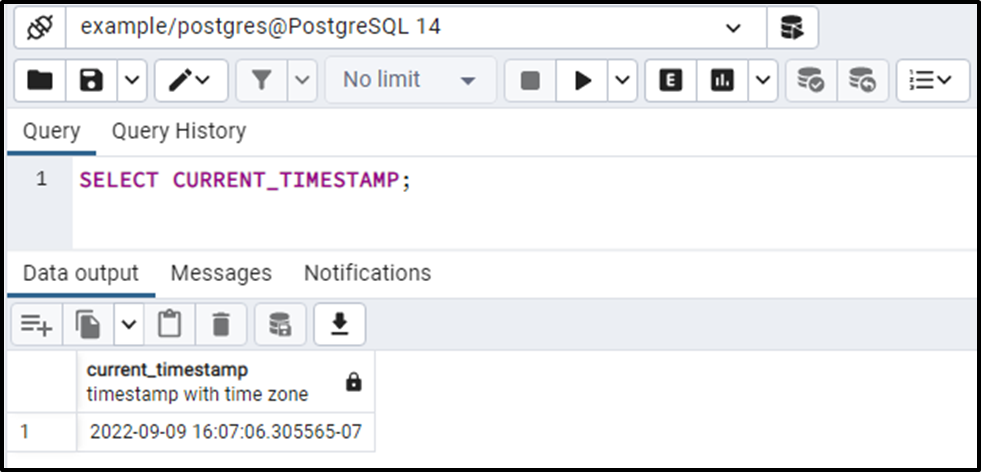The output shows that the CURRENT_TIMESTAMP returns the current date “2022-09-09”, current time “16:07:06.305565” and time zone “07”.

Example #2: How to Use the CURRENT_TIMESTAMP With the Precision Parameter?

In the above example, you can see that there are six digits after the fractional point in the “seconds” field. However, you can pass a value as a precision parameter to round the seconds up to specific fractional points:

`SELECT CURRENT_TIMESTAMP(3);`

In this example, we specified ‘3’ as a precision argument to the CURRENT_TIMESTAMP. Consequently, we will get the following outcome: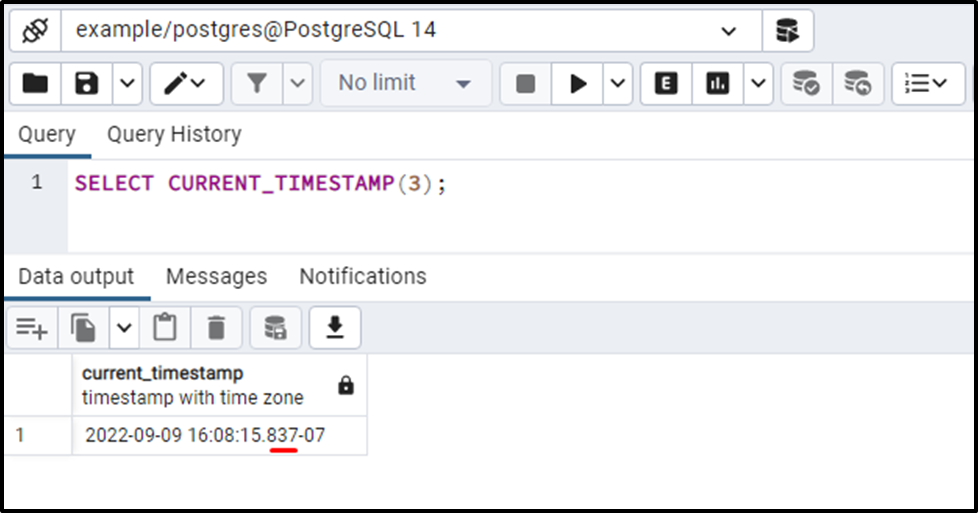The output shows that the seconds are rounded to three decimal points.

Example #3: How to Set Current TIMESTAMP as a Column’s Default Value?

We created a table named publish_article with three columns article_id, article_name, and publish_date:

```CREATE TABLE publish_article(
article_id INT NOT NULL,
article_name TEXT NOT NULL,
publish_date TIMESTAMP WITH TIME ZONE DEFAULT CURRENT_TIMESTAMP);```

In this example, we created a “publish_article” table using the CREATE TABLE command. The table consists of three columns whose details are listed below:

- An article_id column that will accept integer type data.

- An article_name column with the TEXT data type.

- A publish_date column having TIMESTAMP data type. By default, its value would be the current date and time.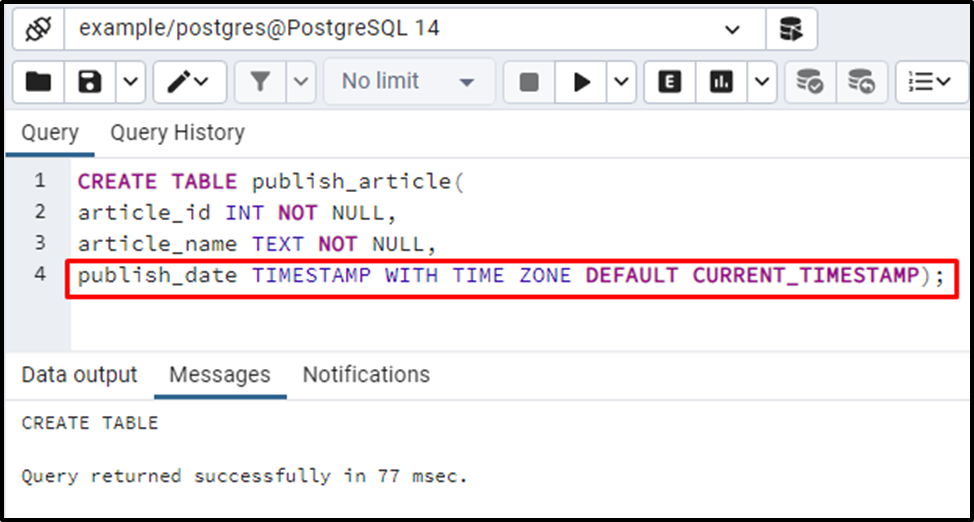The publish_article table has been created successfully. Now, insert the data into the publish_article table with INSERT INTO command:

```INSERT INTO publish_article (article_id, article_name)
VALUES (1, 'PostgreSQL VS MySQL'),
(2, 'What is PostgreSQL');```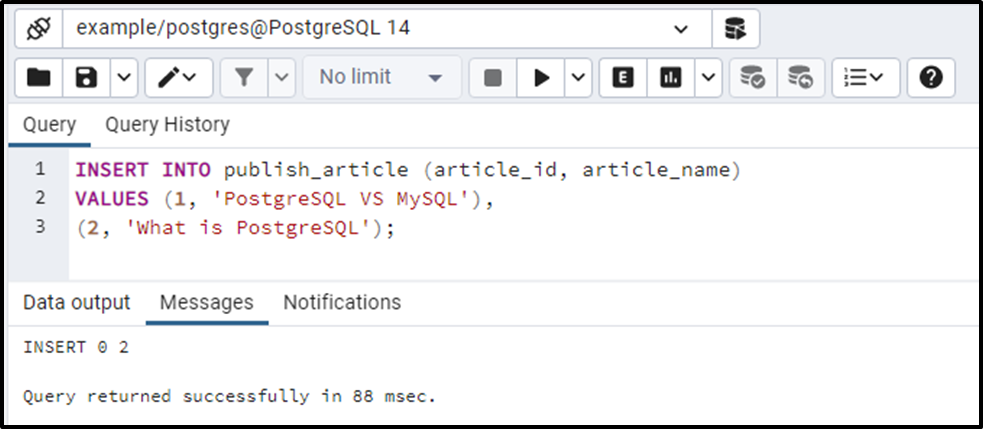Two rows have been inserted into the publish_article table successfully. The above screenshot clears that we didn’t insert any value in the publish_date column. Let’s run the SELECT statement to see the data inserted into the publish_article table:

`SELECT * FROM publish_article;`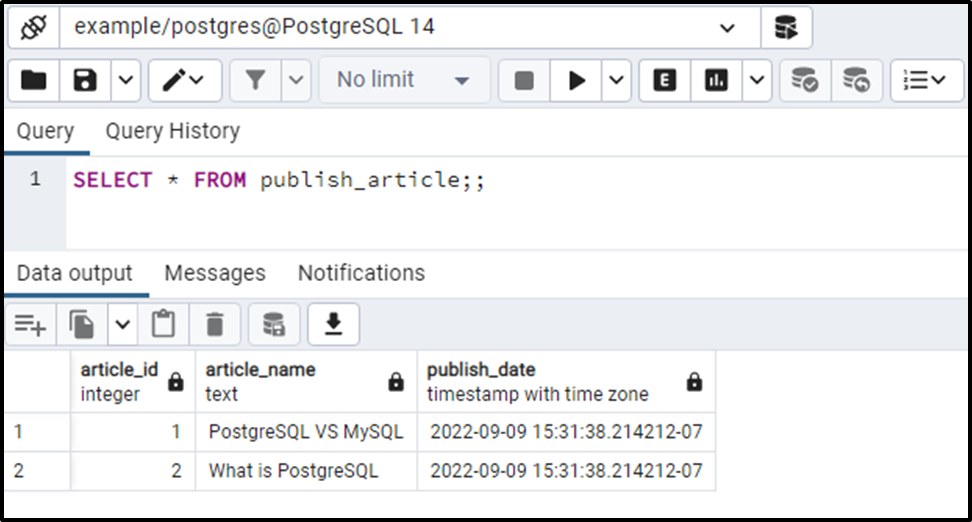By default, Postgres assigns the current date and time to the publish_date column. This way, you can specify the CURRENT_TIMESTAMP as a column's default value.

That was all you needed to learn about the Postgres CURRENT_TIMESTAMP() Function.

Conclusion

The CURRENT_TIMESTAMP function retrieves the current date, time, and timezone when a transaction starts. Round the "seconds" fields to specific fractional digits by passing the "precision" argument to the CURRENT_TIMESTAMP function. However, by omitting the precision parameter, the CURRENT_TIMESTAMP will return the current date, time, and time zone with a full fractional second’s precision. This write-up explained what CURRENT_TIMESTAMP is and how to use it in PostgreSQL with examples.